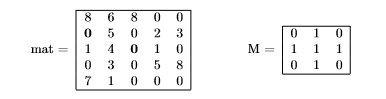# (Solved): Write The C Function Void Matrix_check (int **mat, Int R, Int C, Int **M); Which Receives An Integer...

Write the C function void matrix_check (int **mat, int r, int c, int **M); which receives an integer matrix mat of r rows and c columns, and a matrix M of size (3Ã—3), and it ï¬nds all possible overlapping positions of M with respect to mat such that no elements of mat diï¬€erent from zero overlap with an element of M equal to zero. For each possible overlapping position, the function must print the coordinates of the top-left overlapping element of mat. The candidate must consider only overlapping positions where M is completely included within mat boundaries. Suppose that both r and c are larger than or equal to 3. For example, if mat and M are the following ones:the function must print coordinate pairs (1,0) and (2,2), representing the bold elements

mat = 8 0 1 0 7 6 5 4 3 1 8 0 0 0 0 0 2 1 5 0 0 3 0 8 0 M = 0 1 0 1 1 1 0 1 0

We have an Answer from Expert